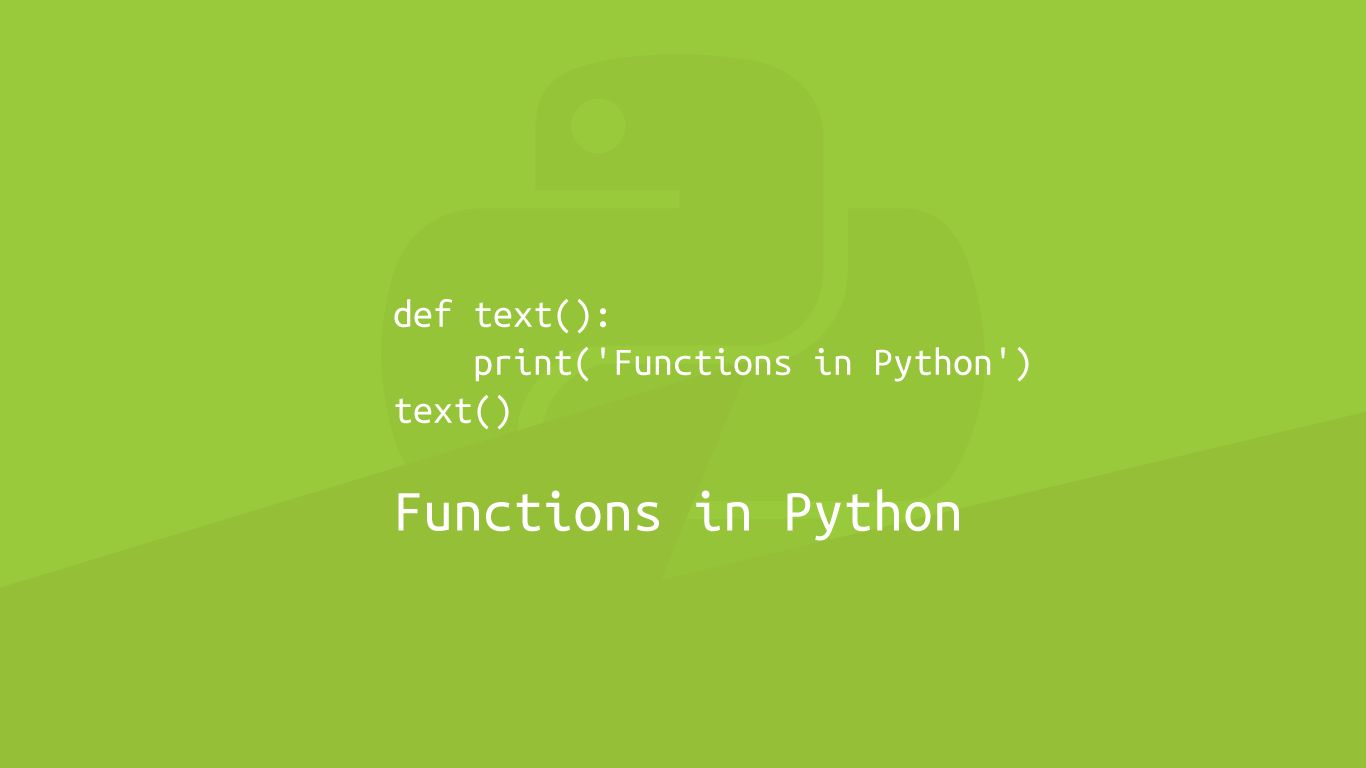# How To Use Functions in PythonFunctions in Python are used to contain blocks of code for performing specific tasks and to contain variables that are locally available to that function. Functions don't run until you call them in the program and are often used as a way to reduce repetition of code.

In this tutorial, we will cover how to use functions in Python from how to define them to the different ways they can be used.

## How to Define a Function

There are a set of components that make up a function in Python:

• `def` - this tells Python to define the following function.
• the name of the function - you can call your function anything you want so long as the name does not contain spaces, special characters or begin with a number.
• the parentheses - `()` (opening and closing brackets) used to pass data into the function
• the arguments - an optional list of variables that are passed into the function inside the `()` (parentheses)
• `:` - a colon to start the actual function

Let's make a function called `text` that will print `hello`.

``````def text():
print('hello')
``````

The code inside the function must be indented with a `    ` (tab), or Python will not consider it to be in the function.

## How to Call a Function

You will have noticed that when you run the above code it does nothing. That is because for a function to run it must be called. To call a function in Python type its name followed by `()` (parenthesis).

``````def text():
print('hello')
text()
``````
``````hello
``````

The function must be called after it is defined in the code. The following example will throw an error.

``````text()
def text():
print('hello')
``````
``````NameError                                 Traceback (most recent call last)
<ipython-input-1-cc344ccaef29> in <module>
----> 1 text()
2 def text():
3    print('hello')

NameError: name 'text' is not defined
``````

## How to Return Data from a Function

To return data from a function, use `return` followed by the data to return. This could be a variable, string, number, list or any other type of data available in Python.

``````def text():
return "hello"

print(text())
``````
``````hello
``````

note - anything placed after `return` in the function will not run.

``````def text():
return "hello"
print('something')

print(text())
``````
``````hello
``````

## Variable Mapping with a Function

Functions in Python can be used to map variables. Let's say we needed to divide a bunch of values by `2` before the value is set as a variable. We could divide the number each time in the code, or we could make a function that would be called every time we wanted to divide something in half and `return` the new value.

``````def divide(num):
return num / 2

a = divide(10)
b = divide(5)

print(a)
print(b)
``````
``````5.0
2.5
``````

The nice thing about using functions like this is if we needed to change its behaviour we just have to edit that one function. It follows the Don’t Repeat Yourself (DRY) software development principle.

## Passing Multiple Arguments into a Function

Multiple arguments can be passed into a function by separating them with a `,` (comma).

``````def divide(num1, num2):
num1 = num1 / 2
num2 = num1 / 2

print(num1)
print(num2)

divide(5, 7)
``````
``````2.5
1.25
``````

## Default Arguments

Arguments can be set to have a default value if one is not supplied in the call. This is done by setting the argument using `=` (equals).

``````def total_fruits(banana, apple, orange, peach = 4):
return banana + apple + orange + peach

bananas = 5
apples = 8
oranges = 9

total = total_fruits(bananas, apples, oranges)

print(total)
``````
``````26
``````

## Variable Length Arguments

If a function has to take an unknown number of arguments, they can can be collected into a `tuple` by putting an `*` (astrix) before the last argument.

``````def total_fruits(banana, *numtuple):
print("first argument: ", banana)
print("tuple: ", numtuple)

bananas = 5
apples = 8
oranges = 9
pears = 4
total_fruits(bananas, apples, oranges, pears)
``````
``````first argument:  5
tuple:  (8, 9, 4)
``````

## Anonymous Functions

It is possible to create discreet anonymous functions with Python. They are also known as lambda functions and the syntax looks like this:

``````lambda arguments: expression
``````

Here is an example of a lambda function to divide a number in half.

``````divide = lambda num: num / 2

half_value = divide(7)
print(half_value)
``````
``````3.5
``````

## Conclusion

You now know how to use functions in Python, why they are help  reduce code repetition and how arguments can be passed in.

#### Related Tutorials### How to Create and Use Lists (Arrays) in Python

September 01, 2020### How to Calculate Mean, Median, Mode and Range in Python

October 07, 2020### How to use the Python filter() Function

September 28, 2020### How to Use Classes and Objects in Python

September 15, 2020### How to Get Inputs in Python

October 01, 2020October 01, 2020